Solution and Gradient Plots with pdeplot and pdeplot3D

To visualize a 2-D scalar PDE solution, you can use the pdeplot function. This function lets you plot the solution without explicitly interpolating the solution. For example, solve the scalar elliptic problem $-\nabla u=1$ on the L-shaped membrane with zero Dirichlet boundary conditions and plot the solution.

Create the PDE model, 2-D geometry, and mesh. Specify boundary conditions and coefficients. Solve the PDE problem.

model = createpde;
geometryFromEdges(model,@lshapeg);
applyBoundaryCondition(model,'dirichlet', ...
'Edge',1:model.Geometry.NumEdges, ...
'u',0);
c = 1;
a = 0;
f = 1;
specifyCoefficients(model,'m',0,'d',0,'c',c,'a',a,'f',f);
generateMesh(model);

results = solvepde(model);

Use pdeplot to plot the solution.

u = results.NodalSolution;
pdeplot(model,'XYData',u,'ZData',u,'Mesh','on')
xlabel('x')
ylabel('y')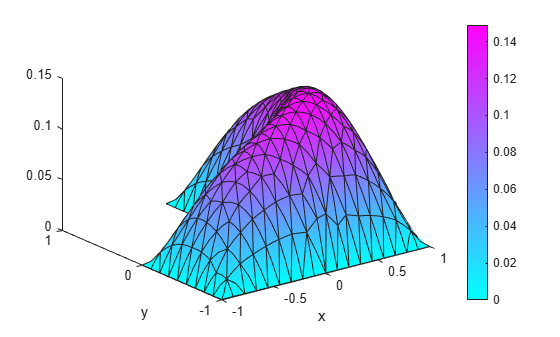To get a smoother solution surface, specify the maximum size of the mesh triangles by using the Hmax argument. Then solve the PDE problem using this new mesh, and plot the solution again.

generateMesh(model,'Hmax',0.05);
results = solvepde(model);
u = results.NodalSolution;

pdeplot(model,'XYData',u,'ZData',u,'Mesh','on')
xlabel('x')
ylabel('y')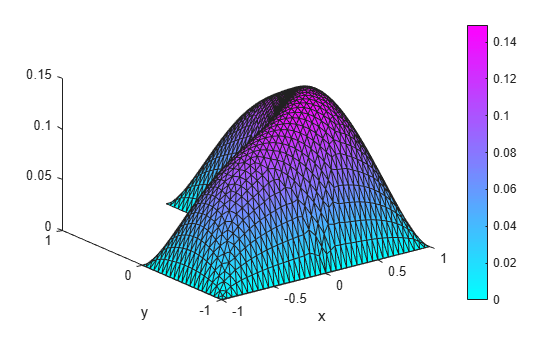Access the gradient of the solution at the nodal locations.

Plot the gradient as a quiver plot.

pdeplot(model,'FlowData',[ux,uy])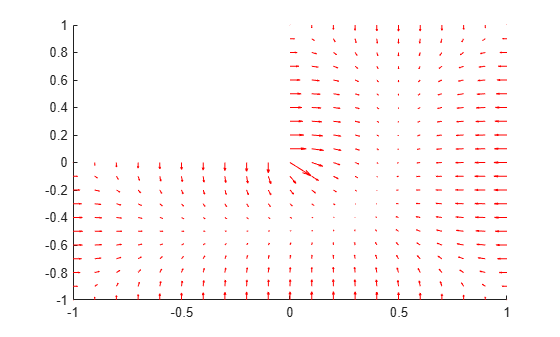Obtain a surface plot of a solution with 3-D geometry and N > 1.

First, import a tetrahedral geometry to a model with N = 2 equations and view its faces.

model = createpde(2);
importGeometry(model,'Tetrahedron.stl');
pdegplot(model,'FaceLabels','on','FaceAlpha',0.5)
view(-40,24)Create a problem with zero Dirichlet boundary conditions on face 4.

applyBoundaryCondition(model,'dirichlet','Face',4,'u',[0,0]);

Create coefficients for the problem, where f = [1;10] and c is a symmetric matrix in 6N form.

f = [1;10];
a = 0;
c = [2;0;4;1;3;8;1;0;2;1;2;4];
specifyCoefficients(model,'m',0,'d',0,'c',c,'a',a,'f',f);

Create a mesh for the solution.

generateMesh(model,'Hmax',20);

Solve the problem.

results = solvepde(model);
u = results.NodalSolution;

Plot the two components of the solution.

pdeplot3D(model,'ColorMapData',u(:,1))
view(-175,4)
title('u(1)')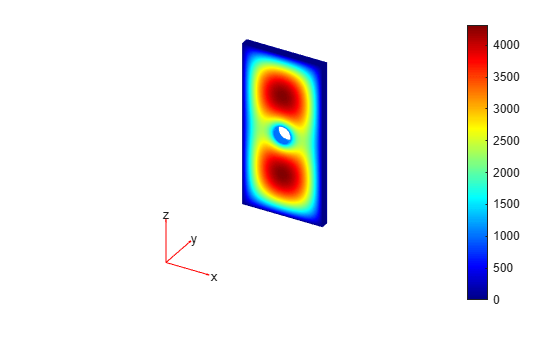figure
pdeplot3D(model,'ColorMapData',u(:,2))
view(-175,4)
title('u(2)')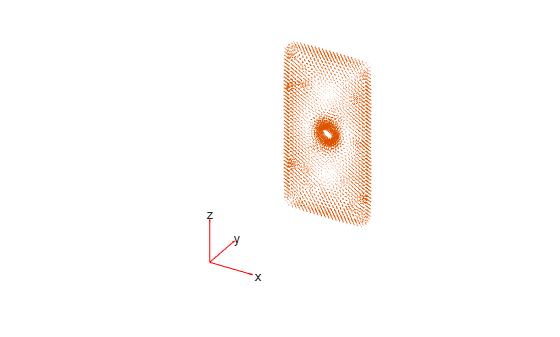Compute the flux of the solution and plot the results for both components.

figurefigure